# Creating a 3D-printable Lorenz attractorThe Lorenz attractor is a set of chaotic solutions of the Lorenz system which, when plotted, resemble a butterfly or figure eight. The Lorenz system is a system of ordinary differential equations first studied by Edward Lorenz in the 1960’s. It is notable for having chaotic solutions for certain parameter values and initial conditions.

In Winter 2015, my colleague Professor Greg Dresden used the Lorenz attractor as an example in his course on Partial Differential Equations. At that time, I worked with Dave Pfaff in the IQ center at W&L to find a way to 3D print a physical model of the solution curves. Dave also created model of the solution curves that could be viewed with 3D glasses in our stereo 3D lab. To create the models we followed three steps:

Step 1:  Create points along the solution path (the Lorenz curve) using Mathematica. Here is the Mathematica code Greg developed for this purpose.

(* Here are the differential equations *)
leqns = {  x'[t] & == -3 (x[t] – y[t]),
y'[t] & == -x[t] z[t] + 28 x[t] – y[t],
z'[t] & == x[t] y[t] – z[t] };
(* Here, I define two paths, p1 and p2, which start at slightly-different initial values. *)
p1 = NDSolveValue[{leqns, x == z == 0, y == 1}, Function[Evaluate[{x[\#], y[\#], z[\#]}]], {t, 0, 30}];
p2 = NDSolveValue[{leqns, x == z == 0.03, y == 1},  Function[Evaluate[{x[\#], y[\#], z[\#]}]], {t, 0, 30}];
(* Let’s look at a plot of these two paths, to verify that they seem correct *)
pic1 = ParametricPlot3D[p1[t], {t, 0, 30}, AxesLabel -> {x, y, z}, PlotStyle -> {Red, Opacity [0.5]}];
pic2 = ParametricPlot3D[p2[t], {t, 0, 30}, AxesLabel -> {x, y, z},
PlotStyle -> {Black, Opacity [0.5]}];
(* Finally, we export them as two separate files *)
tableout1 = Table[p1[t], {t, 0, 30, 0.01}];
tableout2 = Table[p2[t], {t, 0, 30, 0.01}];
Export[“TableOut1.xls”, tableout1]
Export[“TableOut2.xls”, tableout2]

Step 2:  Use Excel to tweak the data into a form we can use.

• Open the files in Excel.
• Insert a column before the three columns of numbers, this is column A. Make the first row 1, and the second row 2 in column A.
• Highlight these two entries in column A, then drag the box down to row 3001. The numbers in column A will automatically fill in the correct numbers.
• Highlight all of the numbers in all four columns. Click on Save As, then save the file as a Tab Delimited Text (.txt) file.

Step 3:  Create the thickened Lorenz curve in Cinema 4D.

• Open Cinema 4D
• Construct the spline with the Lorenz data.
• Left click and hold on the Spline button on the top row, then select the Linear (spline) button. Click once on the view-port to add one point.
• Go to the Object Manager. On the right side, click on the Structure button. You’ll see the Point 0, with X, Y and Z coordinates.
• Click on the File button above the Object Manager. Click on Import ASCII Data… Open the .txt file created above.
•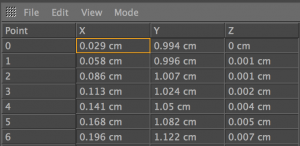Click on the 0th point (the one you added initially) and Delete it. The remaining points are from the Lorenz data (see figure.)
• Click on the Objects button on the right side of the Object Manager.
• Thicken the spline for 3D printing.
• Left click and hold on the Subdivision Surface button on the top menu, then select the Sweep button.
• Left click and hold on the Spline button on the top row, then select the Circle button. Under the Attribute Manager, make the radius of the circle 3mm (0.3cm).
•In the Object Manager, move the Circle and the Spline under the Sweep. The Circle should be above the Spline (see figure). The thickened spline is now complete, and the Lorenz curve can now be exported (as a .stl file) and then 3D-printed.

A thickened Lorenz curve in Cinema 4D is shown below on the left. Two curves can be added to the same plot in Cinema 4D and given different colors.  Dave Pfaff printed two such curves using the Project 260 3D printer at WLU. (This printer uses a gypsum-like powder hardened via a laser then finished with superglue.)  The Project 3D printer can print in color (using inkjet cartridges). Dave also designed a stand for the curves. This gives the beautiful model shown below on the right. Cinema 4D also allows the user to animate the curves. Dave created such an animation, which nicely shows the chaotic nature of the Lorenz attractor. The initial points of the two curves were very close, but in the long term, the curves diverge.

# Five Intersecting Tetrahedra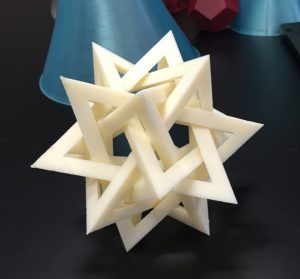One of my favorite mathematical models is the Five Intersecting Tetrhadra model. I first became aware of this model from Thomas Hull, a mathematician at Western New England University. He has written extensively about mathematics and origami (check out his book Project Origami). In particular he has written fantastic instructions for creating this model using modular origami.The five intersecting tetrahedra model is based on the dodecahedron. This one of the five classic regular polyhedra consisting of 12 pentagonal faces and 20 vertices. Take 4 vertices in the dodecahedron which are the same distance apart. These form the 4 vertices of a regular tetrahedron, as shown on the right (figure from Tom). Since the dodecahedron has 20 vertices, we can inscribe 5 such tetrahedra inside the dodecahedron.The origami version comes about by making a thin frame for each tetrahedron. Provided the frames are thin enough, they won’t intersect each other. Instead they will form a marvelous interwoven pattern. As shown on the left, I have successfully followed Tom’s instructions for making the origami version of this model.

The next natural question is whether or not the model can be 3D-printed. The answer, is of course, yes!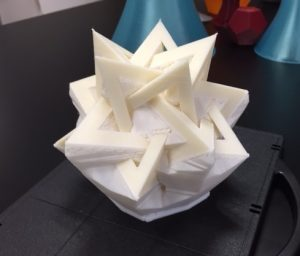I used crsfdr’s model Interlocking Pyramids  from Thingiverse to print the model on the UPrint SE printer at W&L.  The photo at the top shows the finished version. The one to the right shows the model just out of the printer before the supports have been removed. Since the supports are made of a material that dissolves, the UPrint was the perfect printer for the job.

My colleague Professor Michael Bush has used the Five Intersecting Tetrahedra model when teaching introductory Group Theory to undergraduate students. Indeed, this was the motivation for 3D-printing the model as the origami version is not really robust enough to use in a classroom setting. The model is a great tool for discussing the rotational symmetries of the dodecahedron (or its dual the icosahedron). Roughly speaking, the rotational symmetries of the dodecahedron act in a natural way on the five tetrahedra giving a permutation representation of the symmetry group. After some noodling around this allows one to see that this group is the alternating group $$A_5$$. (Michael usually suppresses details about the faithfulness of the representation at this level.)

# More quadratic surfaces

In July it was time to get back to designing and 3D printing some more math models. I had previously seen some beautiful models printed by Dave Pfaff on the Series 1 Pro, using its ability to print in a spiral. I wanted to print a large hyperboloid of one sheet using this printing technique.

So I went back to Cinema4D to design the model. I used the Function tool to make the hyperbola $$\frac{x^2}{4} -\frac{y^2}{9}=1$$, which has asymptotes $$y=\pm \frac{3x}{2}$$. I then used the Lathe tool to revolve the hyperbola around the $$y$$-axis and create a hyperboloid of one sheet. I knew, from the asymptotes, that the surface would have an angle  greater than $$45^\circ$$ angle with the $$xz$$-plane. (Recall that in Cinema 4D, the $$y$$-axis points upwards where the $$z$$-axis usually points in mathematics.) Having a steep angle with the plane is important feature when 3D printing in a spiral. Since there won’t be any supports printed, a steep angle increases the chances of a good print.

At this point I used the surface I had created to make two different kinds of models. For the first model, I simply added a top and bottom to create a closed volume.  For the second model, I thickened the surface using the Extrude tool (2mm), again creating a closed volume. The extruded surface had raised top and bottom rims, which meant the model would not sit flat. So I selected all the point around the top (respectively bottom) rim and brought them down (resp. up) an appropriate distance. Apart from this, this thickened model needed very little doing to it. I then created a third model by adding equations and removing them from the thickened surface. This ended up being quite involved as I used the Bend Tool to make sure the equations sat nicely on the surface.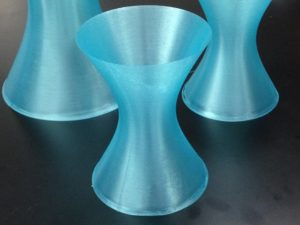In order to get a spiral print, I took the first closed model, and adjusted the print settings on the Series 1 Pro printer. The top and bottom layers of the model are not printed; instead the nozzle goes around in a spiral, printing the surface 1 layer thick. Initially, we had trouble with both the fan and print speed settings. This meant the filament was not setting at the bottom of the model, leaving a gaps and giving a ragged appearance. After we slowed the print speed down and made sure the fan turned on after 1mm (rather than after 10mm), the prints turned out beautifully. The small model is about 13cm tall, and the large one about 20cm tall. These models can be found on Thingiverse here.

The next set of models was the hyperbolic paraboloid again, but this time I wanted to surface to look more like a saddle shape. To achieve this I went into Mathematica and use ParametricPlot3D command to plot the surface $$z=x^2-y^2$$. Instead of displaying it inside a box, I had Mathematica just show the part of the surface inside the circle $$x^2+y^2=1$$. To do this, I used the RegionFunction command.

I exported the Mathematica file as a .wrl to Cinema4D. Once there, I extruded the surface (again 2mm thick), then had to spend a significant amount of time repairing the mesh. To do this, I used the Optimize function, and also went around the surface repairing the mesh by hand (removing points, lines and faces, then using the Fill Polygon Hole tool to repair the gaps). I made two copies of the model, one with equations and one without. (I also built the equations in Cinema4D and extruded them. I then removed them from the surface using the Boole tool.) I 3D printed the resulting models on the FormLabs Form 1+ printer in clear and grey resin. The surfaces printed very well, though there were a fair number of supports to remove.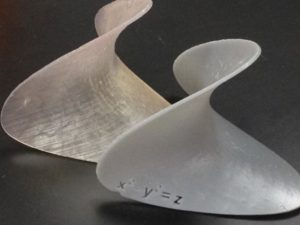These models can be found on Thingiverse here.

# Crocheted Hyperbolic PlanesTwo posts ago, I described my wonderful visit to the Double Helix STEAM school in Athens GA. My goal was to explore hyperbolic planes with the students. They can be hard to understand and visualize, so I thought I’d write another post with some further musings.

Take a piece of paper, and place the it on a flat surface like a table (or wall or board, or something). Notice that each point on the paper is above exactly one point on the table. Now take a ball (or something round – like the top of your head). Try do do the same thing with the paper. What do you notice?

You will notice that the paper needs to be creased in order for it lie smoothly against the ball. If you want to have exactly one point on the paper above one point on the ball, then you will need to remove some paper. The crocheted hyperbolic plane shown above is the exact opposite. There is “too much” plane for the paper. In order to have exactly one point on the paper above one point on the hyperbolic plane, then more paper needs to be added.

It is this idea of removing and adding paper that allows us to construct  paper models which approximate spheres and hyperbolic planes. The plane can be thought of as being tiled by hexagons, like a honeycomb. Now, replace one of the hexagons with a pentagon. If you keep doing this in the right way, you’ll end up with a soccer ball shown on the left below. There, each pentagon is surrounded by hexagons. Since hexagons have been “removed” (in comparison with the planar honeycomb), the surface curves inwards and around creating a spherical shape. If, on the other hand, you replace a pentagon with a heptagon (7-sides) and surround it by hexagons, then “extra” hexagons have been added.  The surface will open up and will approximate a hyperbolic plane, as shown below on the right.

The mathematical notion of intrinsic curvature is what lies behind these shapes. Spheres have positive curvature, planes are flat (zero curvature), and hyperbolic planes have negative curvature.  Many people have written wonderful explanations of these ideas. I’d like to just focus on just one consequence. At the end of this post I’ve included a list of resources where you can read more.

Let’s start by thinking about flat surfaces and traditional Euclidean geometry which we all learned (and forgot!) in school. There we learned that the angle sum of the interior angles of a triangle is $$180^\circ$$. That is $$\angle A+ \angle B + \angle C=180^\circ$$, as shown on the right in the figure below. In order to understand what happens on other surfaces, we need to understand triangles. These are geometric figures where three points, the vertices, have been joined by three straight-lines or geodesics.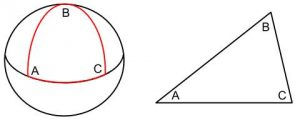On the sphere, it turns out that “straight lines”, or geodesic lines, are sub-arcs of great circles. These are circles formed by the intersection of the sphere with a plane through the center of the sphere. For example, if we think of the earth as a sphere, then the equator and lines of longitudes are all examples of geodesic lines. However the other lines of latitude are not geodesic lines, since they are the intersection of the sphere/earth with planes that do not go through the center of the sphere. In the figure above, you can see a spherical triangle with one side part-way around the equator and the other two from the north pole to the equator. Angles $$\angle A$$ and $$\angle C$$ are both $$90^\circ$$, and $$\angle B$$ is also $$90^\circ$$. This means that $$\angle A + \angle B + \angle C = 270^\circ>180^\circ$$! Indeed, for any spherical triangle the sum of the interior angles is bigger than $$180^\circ$$, that is $$\angle A + \angle B + \angle C >180^\circ$$.

Can you see that the red spherical triangle bounds two areas? (One smaller obvious one, and the larger one on the rest of the sphere.) Can you work out what is the largest possible sum of interior angles of a spherical triangle? It turns out that small spherical triangles approximate planar triangles, so their interior angle sum is close to, but still greater than $$180^\circ$$.What about hyperbolic triangles? Turns out you can create them on a  crochet model by sewing straight lines for the triangle sides, as shown on the left.  Hyperbolic triangles are “skinny”; the sum of the interior angles is less than $$180^\circ$$. In the triangle shown below, you can clearly see the interior angles are small and their sum is much less than $$180^\circ$$. As in the spherical case, small hyperbolic triangles are approximately planar; here the interior angle sum is close to, but still smaller than $$180^\circ$$. Amazingly, the area of hyperbolic triangles whose vertices approach infinity is finite. The proof of this result can be found in any undergraduate textbook on hyperbolic geometry.I promised to include some references, and here they are.

Two of my favorite undergraduate texts include:

Post Script: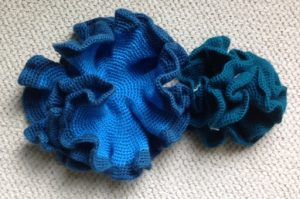When crocheting these hyperbolic planes I weighed out three balls of the same yarn in different colors. Since the length of yarn was approximately the same, the number of crochet stitches were approximately the same, and hence the area made was the same.  Pretty amazing to think that the blue area and the green area around the edge are the same! It just shows how much extra fabric is created as the hyperbolic plane is crocheted.

# Flowers everywhereIn early May, the IQ center at W&L was filled with 3D-printed flowers. Dave Pfaff and his work study students printed flowers in many bright colors on the Cura 3D printer. They are from Super Flowers found on Thingiverse. The fine filaments are created by printing in the air. That’s right, the printer puts down a single layer of material, then returns to the center. Since there are no supports underneath, a “droop-loop” of filament is created.The vases were also designed by Dave Pfaff. He started with a flower shape on the base, then expanded and twisted the shape around creating the vase shape we see. Fabulous work!

# Visiting Double Helix STEAM school.At the end of April I headed down to Athens Georgia to visit my friend and collaborator Professor Jason Cantarella at the University of Georgia, Athens. While there I had the opportunity to visit the Double Helix STEAM school and talk with the students about Different kinds of geometry, in particular hyperbolic planes.We started by discussing planes, spheres and the hyperbolic crocheted planes I bought. We talked about triangles and the interior angle sums of the different triangles ($$180^\circ, \, >180^\circ,\, <180^\circ$$ respectively).The students found the idea of great circles and spherical triangles pretty mind blowing, especially when I gave them an example of a triangle with three $$90^\circ$$ angles. However, they were quickly on board and immediately suggested spherical triangles with even larger internal angle sums.We then talked about the way a plane can be tiled with hexagons, and then how a soccer ball is made by switching out a hexagon (6-gon) with a pentagon (5-gon).  Since there is less material, the surface has the positive curvature of a sphere. A model of a hyperbolic plane can be made when a hexagon is replaced with a heptagon (7-gon).  The extra material gives the negative curvature of the hyperbolic plane.The Institute for Figuring has some great information about this model and has instructions for building one too. We used these instructions to build our own hyperbolic planes.  The students used scissors and sticky tape to construct their models. They were awesome!

# Museum MagicRecently, I had a wonderful two days visiting Ricardo Nemirovsky and his team in San Diego. I started by visiting the wonderful Fleet Science Center and being the mathematician for their Meet the Mathematician event on the Sunday afternoon. (I even got my own poster – wow!) Ashanti Davis met me and showed me around the Taping Shape* exhibit for which I had designed the 3D-printed mathematical models.These photos don’t really capture how marvelous it was to walk inside the topological shapes. How often does a mathematician get to explore the interior of a torus, or walk down the leg of a pair of pants?!? The lighting kept changing color as well, adding to the experience.

I was able to see my 3D-printed models in action; anything from little kids throwing them around, to big kids and grandparents building more complex topological shapes.The folks at the museum had set up a tank with soapy water, and the frame for the Schwarz P surface could be lowered into and out of the soapy water by folks using a wheel. The frame worked perfectly, beautifully showing the Schwarz P surface.

I spent my hours in the museum talking with people who stopped by about the math of the Taping Shape exhibit and of the 3D printed models. Using zome tools and another tank of soapy water, I was able to demonstrate just a few of the many different shapes that soap film (a.k.a. minimal surfaces) take. The kids experimented with their own zome tool shapes. They created some bizarre models giving interesting soap films. Many of the soap films we created showed the classic angles where three or more soap films joined together. (Math in action really does work!) It was a wet and fun time, and my hands ended up very, very clean. Thanks go to Ashanti for setting things up and keeping me company during much of the afternoon.A number of my designs had been 3D-printed into giant sized models, which was great to see. On the final day of my visit I was able to meet with the entire Informath team. I was also able to hang out with Bohdan Rhodehamel and see his lab. He was responsible for 3D-printing and then assembling the models for the Taping Shape exhibit. I wrapped up my trip by giving the math department’s colloquium on Mathematics and 3D Printing at San Diego State University.

*The Taping Shape exhibit is part of the InforMath project funded by the National Science Foundation (DRL-1323587).  (The InforMath Project is a partnership between San Diego State University and several museums at the Balboa Park, including the Rueben H. Fleet Science Center .)

# Knots as ribbons

I’ve continued with my project to edit the 3D printed models my Fall 2014 Math 341 Introduction to Topology class made. Recently, I came across two of my favorite pieces. The first is a model that was designed by Emily Jaekle (’16) and is a ribbon version of the (3,5) torus knot.

This $$(5,3)$$ ribbon torus knot was designed entirely in Cinema4D. The curve was created using the Formula tool with parametrization $$x(t)=(2+\cos(5t))\cos(3t), y(t)=(2+\cos(5t))\sin(3t), z(t)=-\sin(5t)$$ for $$t\in[-\pi, \pi]$$. The trianglulated surface was created by first adding in a small rectangle, then using the SweepNurbs (without caps). The rectangle was also rotated 1800 degrees in the process. The small gap was fixed using the Bridge tool in Edge mode. This ribbon knot was originally printed in blue on the Projet-260 3D Systems printer. Later, I printed it on the FormLabs 1+ printer in black resin. You can find the model here on Thingiverse.

The second model was designed by Cathy Wang (’15) and is a ribbon version of the (3,2) trefoil knot with an amazing color scheme.The entire model was designed in Cinema4D. The knot was created using the Formula tool with parametrization $$x(t)=(2+\cos(2t))\cos(3t), y(t)=(2+\cos(2t))\sin(3t), z(t)=-\sin(2t)$$ for $$t\in[-\pi,\pi]$$. The trianglulated surface was created by first adding in a small rectangle, then using the SweepNurbs (without caps). The width and height of the rectangle was adjusted so the band is not a constant size through the knot. The overlapping edges and small gaps were also fixed. Finally, the knot was colored with a beautiful rainbow-gradient. This ribbon knot was originally printed in rainbow colors on the Projet-260 3D Systems printer. Later, I printed it on the FormLabs 1+ printer in black resin. You can find the model here on Thingiverse.

# Knots, a Seifert Surface, and an Octopus.

I’ve recently been going back to the 3D printed models my students designed in my Math 341 Introduction to Topology class in Fall 2014. I know so much more now than I did then when I first started on this journey. So, I decided to go back to the designs, check over them, edit them (as necessary), then reprint them and publish them on Thingiverse.

The first two models I looked at where Candace Bethea’s (’15) $$6_2$$ knot and Hayley Archer-McClelland’s (’15) three interlocking trefoils. In a previous class using 3D printing, Professor Aaron Abrams contacted the developer of the program Seifert View, and arranged for the computed curves and surfaces to be able to be exported as a file. (This free software normally doesn’t allow you to do this.) We have since found this to be an invaluable tool for 3D printing knots and knots with Seifert surfaces.

The $$6_2$$ knot was first adjusted in, then exported from SeifertView, and then opened in Cinema4D. The surface of the knot needed fixing due to overlapping polygons. This was fixed by deleting overlapping polygons, then filling in the gaps using the Stitch and Sew tool in Edge mode. The knot was originally printed in orange on the Projet-260 3D Systems printer. Later, I printed it on the FormLabs 1+ printer in clear resin. You can find the model here on Thingiverse.

The three interlocking trefoils were designed entirely in Cinema4D. The parametric equations of a trefoil knot are $$x(t)= (2+\cos(3t))\cos(2t), y=(2+\cos(3t))\sin(2t), z=-\sin(3t)$$ for $$t\in[-\pi, \pi]$$. We first made the curve with the Formula tool for $$t\in[-3.14, 3.14]$$. We added a SweepNurb (with no caps) consisting of a circle with radius 0.2 cm around the curve. The choice of $$t$$ meant there was a small gap between the ends of the tube. We again sealed this gap using the Stitch and Sew tool in Edge mode. The knots were originally printed in pink, pale green and blue on the Projet-260 3D Systems printer. Later, I printed it on the FormLabs 1+ printer in clear resin. You can find the model here on Thingiverse.

Mithra Muthukrishnan (’16) used Seifert View to design a figure-8 knot and its Seifert surface. After tweaking in Seifert View, she exported the surface to Cinema4D. It ended up being a complex design process, since we wanted to color the knot and two sides of the surface with different colors. There, the surface was extruded in both directions creating the two sides of the Seifert surface. Finally, three copies of the surface were made. In one, all the surfaces were deleted leaving the knot. In another the knot and one side of the extrusion was deleted. In the final copy, the knot and the other side of the extrusion was deleted. This left three pieces which could each be given their own color before printing. The knot was colored red/pink, the different sides of the Seifert surface were colored white and yellow. The model was originally printed in color on the Projet-260 3D Systems printer. Later, I printed it on the MakerBot 2x printer in bright white. You can find the model here on Thingiverse.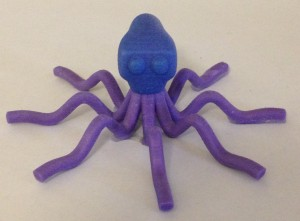The octopus model was designed by DanJoesph Quijada (’15) entirely in Cinema4D. The legs were made using parametric equations like $$x(t) = a\sqrt{2}t, y(t)=0, z(t) = b^2 cos^2(ct)e^{-dt^2}$$ for various constants $$a, b, c,$$ and $$d$$, and bounded time $$t$$. These curves were then thickened using a SweepNurbs. To make the octopus head, we first made a box, then extruded parts of the sides to alter the shape, then applied the Subdivision Surface tool to it. Finally we made minor adjustments, such as adding the eyes and changing the dimensions of the octopus to make it look more realistic. The model was originally printed in pink on the Projet-260 3D Systems printer. Later, I printed it on the MakerBot 2x printer in bright white, though I had some trouble with the legs. You can find the model here on Thingiverse.

# Soap film frame for the Schwarz P surface

In an earlier post on the mathematics of the Schwarz P surface, we saw how minimal surfaces can be understood by viewing them as soap films. The final challenge was to construct a 3D printed soap film frame for the Schwarz P surface for the Taping Shape*  exhibit at the Rueben H. Fleet Science Center.

From the way the Schwarz P surface is constructed, we know the boundaries of the 4-gons lie in the surface. Thus the surface has many straight lines lying in it. There are also many circles (really almost circles) lying in the surface.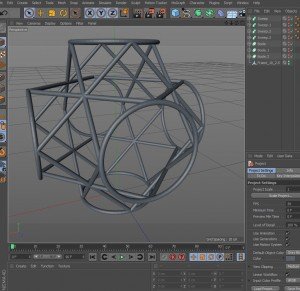To construct a soap film frame in Cinema4D, I simply took these lines and circles and thickened them to get the frame. To prevent interior intersections of the tubes, I used the Boole tool ($$A\cup B$$) as I added in the lines and circles. In essence, this takes the “skin” of the two surfaces and ignores what is inside. The last time I used the Boole tool the surface vanished – it was too much for the program to render. However, by deselecting the High Quality option in the Boole options we were able to get the model to appear. I made three sizes of models: 6cmx6cmx6cm,10cmx10cmx10cm, and 15cmx15cmx15cm. I also made these sizes with two different tube diameters: 2.5mm and 3mm.

Printing the model was another question entirely. We had many fails (two shown below) before we figured out how to print the frame.

In essence, the unsupported parts of the model vibrate when the printer’s extruder is going over them. This leads to the frame being “fuzzy”, and even having visible jumps at some points. The solution was relatively simple. When printing, we selected to have the tubes print as a solid, and we also made sure the entire model had supports. The photo below on the left shows the supports for the uPrint SE print, the one on the right shows the 6cm size 3mm diameter frame printed by the MakerBot 2X replicator.

We found that when we dipped model in soapy water, the soap film gave a lovely approximation of the Schwarz P surface.The frame has a lot of symmetry too. There are many interesting viewpoints, for example as shown on the right by a 10cm size 2.5mm diameter print by the uPrint SE. You can find the files for the model here on Thingiverse.

*The Taping Shape exhibit is part of the InforMath project funded by the National Science Foundation (DRL-1323587).  (The InforMath Project is a partnership between San Diego State University and several museums at the Balboa Park, including the Rueben H. Fleet Science Center .)